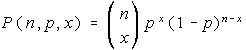BINOMIAL Program

Computes a binomial probability. Requests the values of n, p, and x, and then for the type of inequality. The five different inequalities (equalities) may be chosen: =, <, >, <=, or >=.

The inequalities involve finding several binomial probabilities and summing them.

Formulas